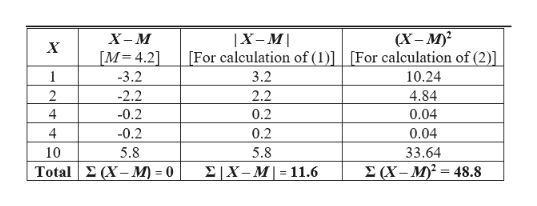# EXAMPLE 4.9Students who seek therapy at university counseling centers often do not attend many sessions. For example, in one study, the median number of therapy sessions was 3 and the mean was 4.6 (Hatchett, 2003). Let’s examine the spread of fictional scores for a sample of five students: 1, 2, 4, 4, and 10 therapy sessions, with a mean of 4.2. We find out how far each score deviates from the mean by subtracting the mean from every score. First, we label with an X the column that lists the scores. Here, the second column includes the results we get when we subtract the mean from each score, or X − M. We call each of these a deviation from the mean (or just a deviation)—the amount that a score in a sample differs from the mean of the sample.XX − M  1− 3.2  2− 2.2  4− 0.2  4− 0.210    5.8But we can’t just take the mean of the deviations. If we do (and if you try this, don’t forget the signs—negative and positive), we get 0—every time. Are you surprised? Remember, the mean is the point at which all scores are perfectly balanced. Mathematically, the scores have to balance out. Yet we know that there is variability among these scores. The number representing the amount of variability is certainly not 0!When we ask students for ways to eliminate the negative signs, two suggestions typically come up: (1) Take the absolute value of the deviations, thus making them all positive, or (2) square all the scores, again making them all positive. It turns out that the latter, squaring all the deviations, is how statisticians solve this problem. Once we square the deviations, we can take their average and get a measure of variability. Later (using a beautifully descriptive term created by our students), we will “unsquare” those deviations in order to calculate the standard deviation

Question

#### EXAMPLE 4.9

Students who seek therapy at university counseling centers often do not attend many sessions. For example, in one study, the median number of therapy sessions was 3 and the mean was 4.6 (Hatchett, 2003). Let’s examine the spread of fictional scores for a sample of five students: 1, 2, 4, 4, and 10 therapy sessions, with a mean of 4.2. We find out how far each score deviates from the mean by subtracting the mean from every score. First, we label with an X the column that lists the scores. Here, the second column includes the results we get when we subtract the mean from each score, or X − M. We call each of these a deviation from the mean (or just a deviation)—the amount that a score in a sample differs from the mean of the sample.

X X − M
1 − 3.2
2 − 2.2
4 − 0.2
4 − 0.2
10     5.8

But we can’t just take the mean of the deviations. If we do (and if you try this, don’t forget the signs—negative and positive), we get 0—every time. Are you surprised? Remember, the mean is the point at which all scores are perfectly balanced. Mathematically, the scores have to balance out. Yet we know that there is variability among these scores. The number representing the amount of variability is certainly not 0!

When we ask students for ways to eliminate the negative signs, two suggestions typically come up: (1) Take the absolute value of the deviations, thus making them all positive, or (2) square all the scores, again making them all positive. It turns out that the latter, squaring all the deviations, is how statisticians solve this problem. Once we square the deviations, we can take their average and get a measure of variability. Later (using a beautifully descriptive term created by our students), we will “unsquare” those deviations in order to calculate the standard deviation

check_circle

Step 1

Note:

Hi there! Thank you for posting the question. However, it is not exactly clear what needs to be found, from the manner in which the question is presented. So, we have solved it to the best of our abilities, by assuming that (1) represents the Mean Absolute Deviation (MAD), and (2) represents the Standard Deviation (SD).

Step 2

Calculation:

Consider the following table for th...help_outlineImage Transcriptionclose(X — М)? [For calculation of (1)] [For calculation of (2)] X-М |X-M M 4.2] 3.2 -3.2 10.24 2.2 2 2.2 4.84 4 -0.2 0.2 0.04 0.04 4 -0.2 0.2 5.8 10 5.8 33.64 2 (X-M)= 48.8 Total(X-M) = 0 |X-M|= 11.6 fullscreen

### Want to see the full answer?

See Solution

#### Want to see this answer and more?

Solutions are written by subject experts who are available 24/7. Questions are typically answered within 1 hour.*

See Solution
*Response times may vary by subject and question.
Tagged in

### Data